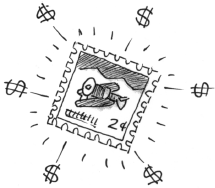### Home > APCALC > Chapter 8 > Lesson 8.1.1 > Problem8-9

8-9.

The value of a rare stamp was $250$ in 1989. The stamp has increased in value by $12\%$ each year.

1. What was the stamp worth in the year 2000?

The stamp is $112\%$ of its value from the previous year.This will happen for $11$ years in a row.

$250·1.12^{11} = 869.64$

2. What was the average value of the stamp from 1989 to 2000?

If $f(t)$ represents the value of the stamp as a function of time (the equation you used to solve part (a)):

$\text{average value of }f(t)=\frac{1}{11}\int_{0}^{11}f(t)dt$

$\frac{1}{11}\int_0^{11}250(1.12)^{x}dx=\left.\frac{1}{11}(250)\frac{1}{\ln(1.12)}(1.12)^x\right|_0^{11}$